# 学生表 成绩表 课程表 教师表postgreSQL 同时被 2 个专栏收录2 篇文章 0 订阅

Student(s_id,s_name,s_birth,s_sex) –学生编号,学生姓名, 出生年月,学生性别

Course(c_id,c_name,t_id) – –课程编号, 课程名称, 教师编号

Teacher(t_id,t_name) –教师编号,教师姓名

Score(s_id,c_id,s_s_score) –学生编号,课程编号,分数

ps：这些题考察SQL的编写能力，对于这类型的题目，需要你先把4张表之间的关联关系搞清楚了，最好的办法是自己在草稿纸上画关联图，然后再编写对应的SQL语句就比较容易了。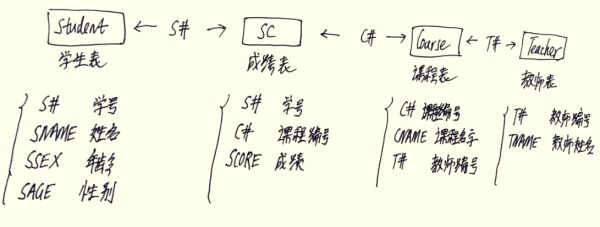–1.学生表
Student(s_id,s_name,s_birth,s_sex) –学生编号,学生姓名, 出生年月,学生性别

–2.课程表
Course(c_id,c_name,t_id) – –课程编号, 课程名称, 教师编号

–3.教师表
Teacher(t_id,t_name) –教师编号,教师姓名

–4.成绩表
Score(s_id,c_id,s_score) –学生编号,课程编号,分数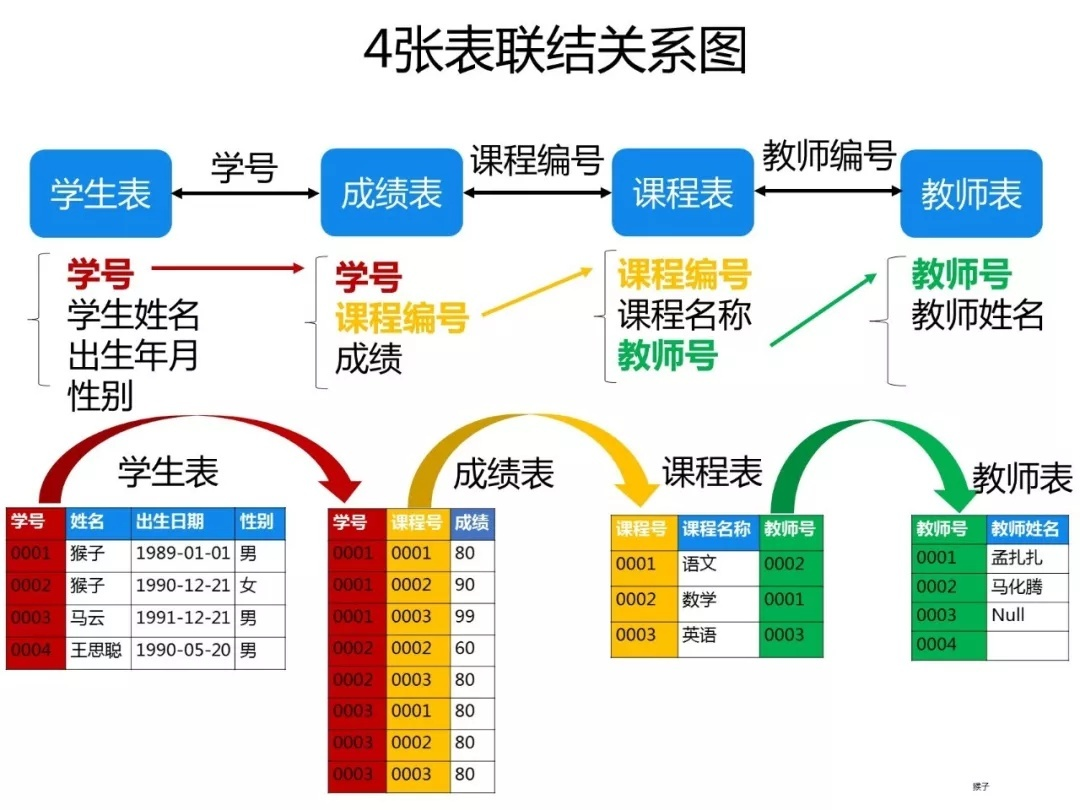## 建表和插入数据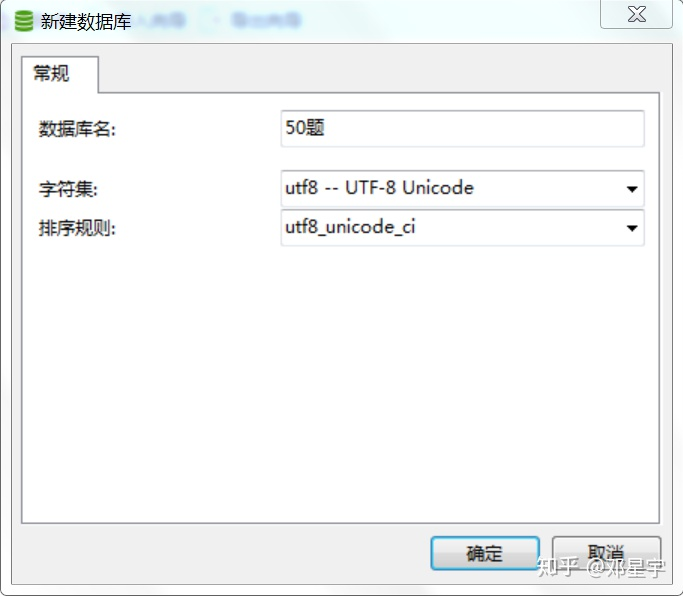-- 学生表
-- Student（s_id，s_name，s_birth，s_sex）
-- 学生编号,学生姓名, 出生年月,学生性别
CREATE TABLE Student(
s_id VARCHAR(20),
s_name VARCHAR(20) NOT NULL DEFAULT '',
s_birth VARCHAR(20) NOT NULL DEFAULT '',
s_sex VARCHAR(10) NOT NULL DEFAULT '',
PRIMARY KEY(s_id)
);
-- 课程表
-- Course(c_id,c_name,t_id)
-- 课程编号, 课程名称, 教师编号
CREATE TABLE Course(
c_id VARCHAR(20),
c_name VARCHAR(20) NOT NULL DEFAULT '',
t_id VARCHAR(20) NOT NULL,
PRIMARY KEY(c_id)
);
-- 教师表
-- Teacher(t_id,t_name)
-- 教师编号,教师姓名
CREATE TABLE Teacher(
t_id VARCHAR(20),
t_name VARCHAR(20) NOT NULL DEFAULT '',
PRIMARY KEY(t_id)
);
-- 成绩表
-- Score(s_id,c_id,s_s_score)
-- 学生编号,课程编号,分数
CREATE TABLE Score(
s_id VARCHAR(20),
c_id VARCHAR(20),
s_score INT(3),
PRIMARY KEY(s_id,c_id)
)

-- 学生表数据
insert into Student values('01' , '赵雷' , '1990-01-01' , '男');
insert into Student values('02' , '钱电' , '1990-12-21' , '男');
insert into Student values('03' , '孙风' , '1990-05-20' , '男');
insert into Student values('04' , '李云' , '1990-08-06' , '男');
insert into Student values('05' , '周梅' , '1991-12-01' , '女');
insert into Student values('06' , '吴兰' , '1992-03-01' , '女');
insert into Student values('07' , '郑竹' , '1989-07-01' , '女');
insert into Student values('08' , '王菊' , '1990-01-20' , '女');
-- 教师表数据
insert into Teacher values('t01' , '张三');
insert into Teacher values('t02' , '李四');
insert into Teacher values('t03' , '王五');
-- 课程表数据
insert into Course values('01' , '语文' , '02');
insert into Course values('02' , '数学' , '01');
insert into Course values('03' , '英语' , '03');

-- 成绩表数据
insert into Score values('01' , '01' , 80);
insert into Score values('01' , '02' , 90);
insert into Score values('01' , '03' , 99);
insert into Score values('02' , '01' , 70);
insert into Score values('02' , '02' , 60);
insert into Score values('02' , '03' , 80);
insert into Score values('03' , '01' , 80);
insert into Score values('03' , '02' , 80);
insert into Score values('03' , '03' , 80);
insert into Score values('04' , '01' , 50);
insert into Score values('04' , '02' , 30);
insert into Score values('04' , '03' , 20);
insert into Score values('05' , '01' , 76);
insert into Score values('05' , '02' , 87);
insert into Score values('06' , '01' , 31);
insert into Score values('06' , '03' , 34);
insert into Score values('07' , '02' , 89);
insert into Score values('07' , '03' , 98);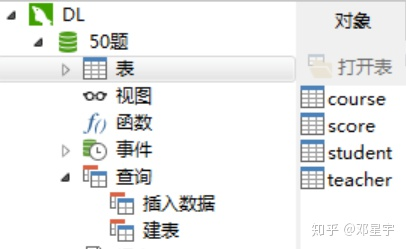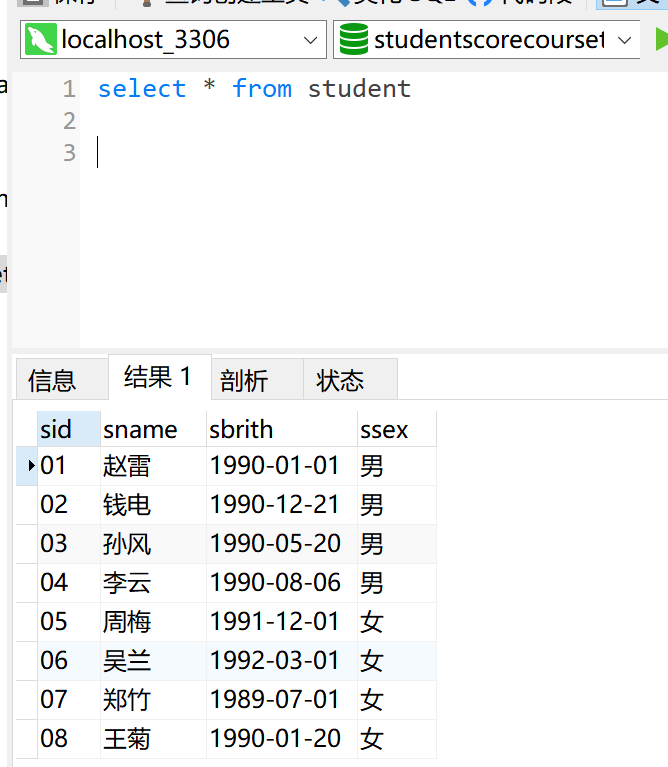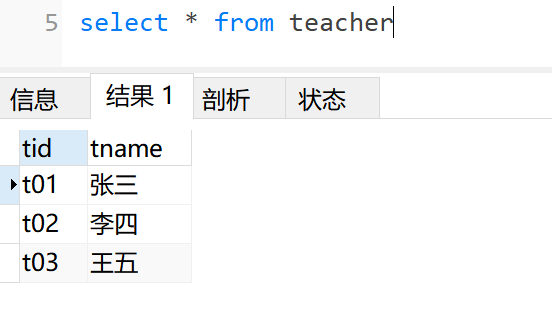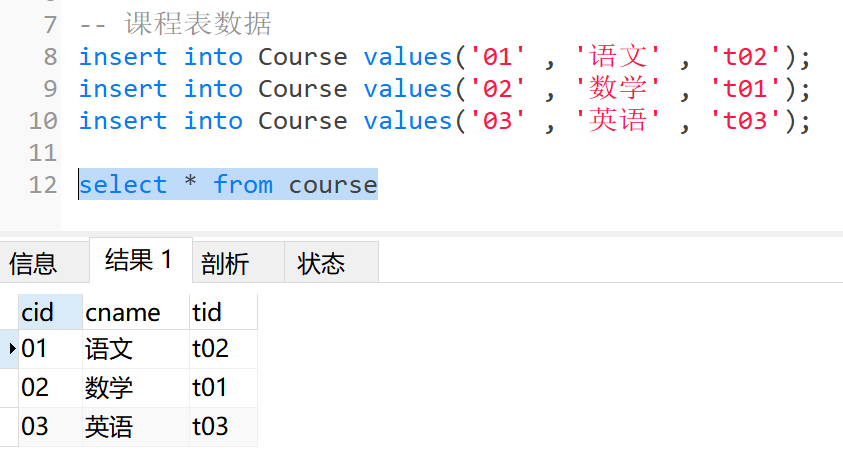## 题目

-- 1.查询课程编号为‘01’的课程比‘02’的课程成绩高的所有学生的学号、姓名和各自‘01’‘02’课程成绩

SELECT a.s_id ,c.s_name,a.s_score '01',b.s_score '02'FROM
(SELECT s_id,c_id,s_score FROM score WHERE c_id='01')AS a
INNER JOIN
(SELECT s_id,c_id,s_score FROM score WHERE c_id='02')AS b ON a.s_id=b.s_id
INNER JOIN student AS c ON a.s_id=c.s_id
WHERE a.s_score>b.s_score;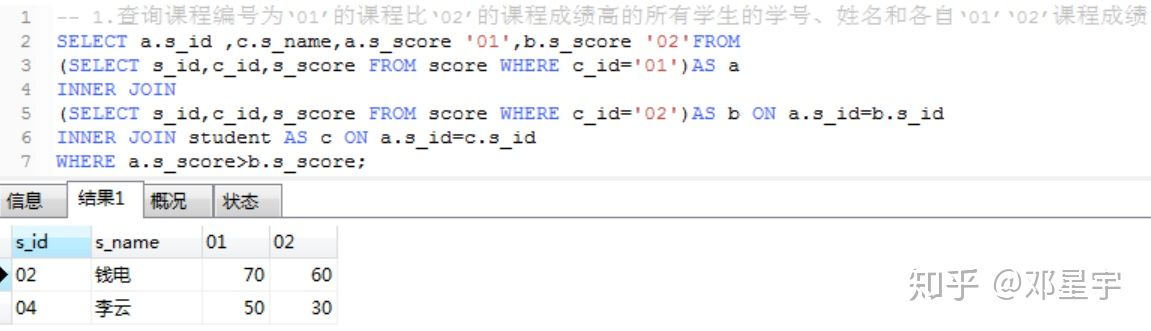-- 2、查询平均成绩大于60分的学生的学号和平均成绩

SELECT s_id,AVG(s_score)
FROM score
GROUP BY s_id HAVING AVG(s_score)>60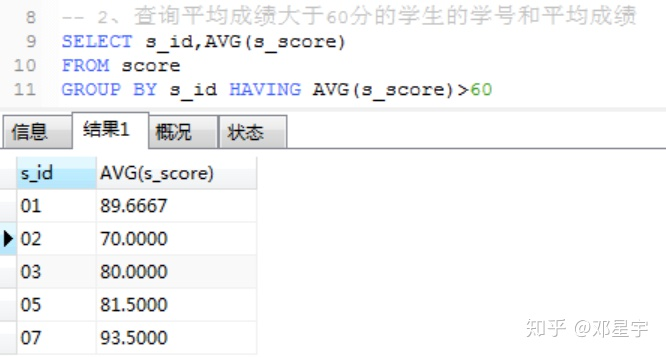-- 3、查询所有学生的学号、姓名、选课数、总成绩

SELECT a.s_id,a.s_name,COUNT(b.c_id),SUM(IFNULL(b.s_score,0))
FROM student AS a
LEFT JOIN score AS b
ON a.s_id=b.s_id
GROUP BY s_id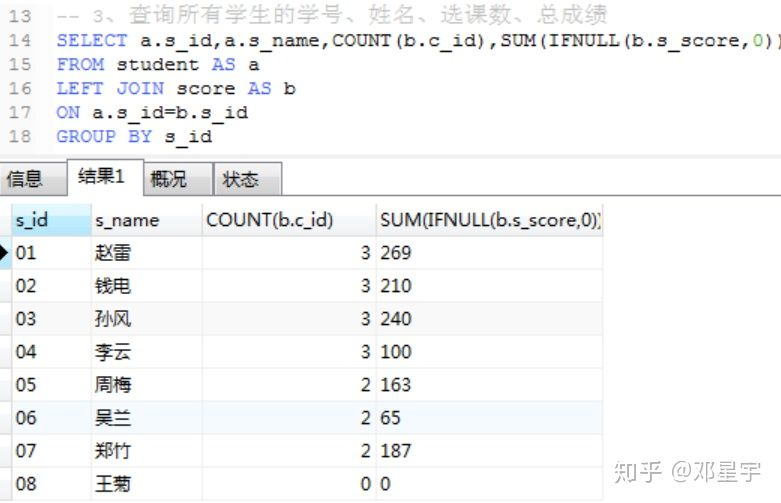-- 4、查询姓“张”的老师的个数

SELECT COUNT(t_id)
FROM teacher
WHERE t_name LIKE '张%'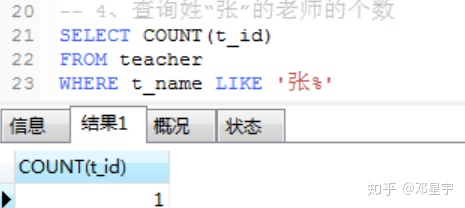-- 5、查询没学过“张三”老师课的学生的学号、姓名

SELECT s_id,s_name FROM student
WHERE s_id NOT IN(
SELECT s_id FROM score
WHERE c_id =
(SELECT c_id FROM course
WHERE t_id=
(SELECT t_id FROM teacher
WHERE t_name = '张三')))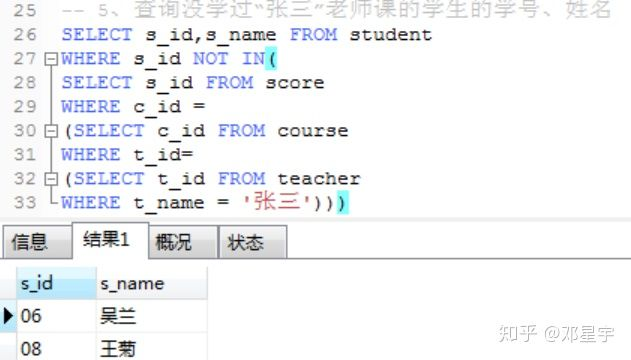SELECT s_id,s_name FROM student
WHERE s_id NOT IN(
SELECT a.s_id FROM score AS a
INNER JOIN course AS c ON c.c_id=a.c_id
INNER JOIN teacher AS d ON d.t_id=c.t_id
WHERE d.t_name='张三')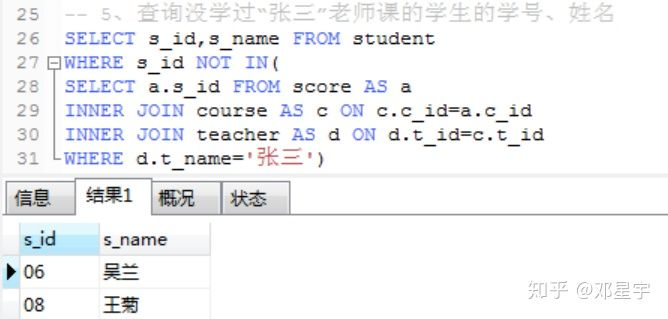-- 6、查询学过“张三”老师所教的所有课的同学的学号、姓名

SELECT st.s_id,st.s_name FROM student AS st
INNER JOIN score AS s ON st.s_id=s.s_id
INNER JOIN course AS c ON s.c_id=c.c_id
INNER JOIN teacher AS t ON t.t_id=c.t_id
WHERE t.t_name ='张三'
GROUP BY st.s_id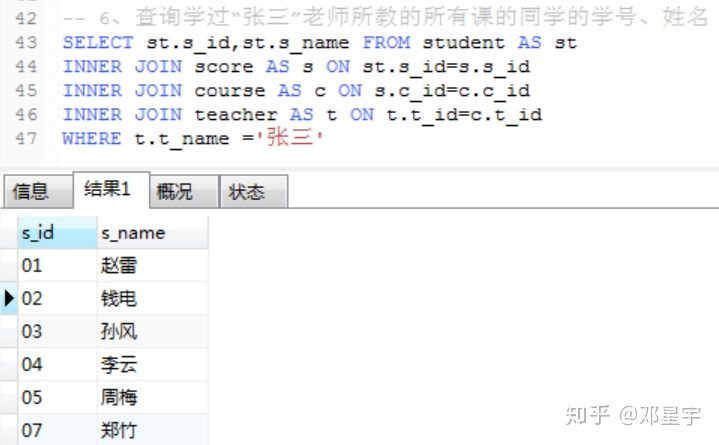SELECT st.s_id,st.s_name FROM student AS st
INNER JOIN score AS s ON st.s_id=s.s_id
INNER JOIN course AS c ON s.c_id=c.c_id
INNER JOIN teacher AS t ON t.t_id=c.t_id
WHERE t.t_name ='张三'
GROUP BY st.s_id
HAVING
COUNT(st.s_id) IN
(SELECT COUNT(c_name) FROM course
INNER JOIN teacher
ON course.t_id=teacher.t_id
WHERE teacher.t_name='张三')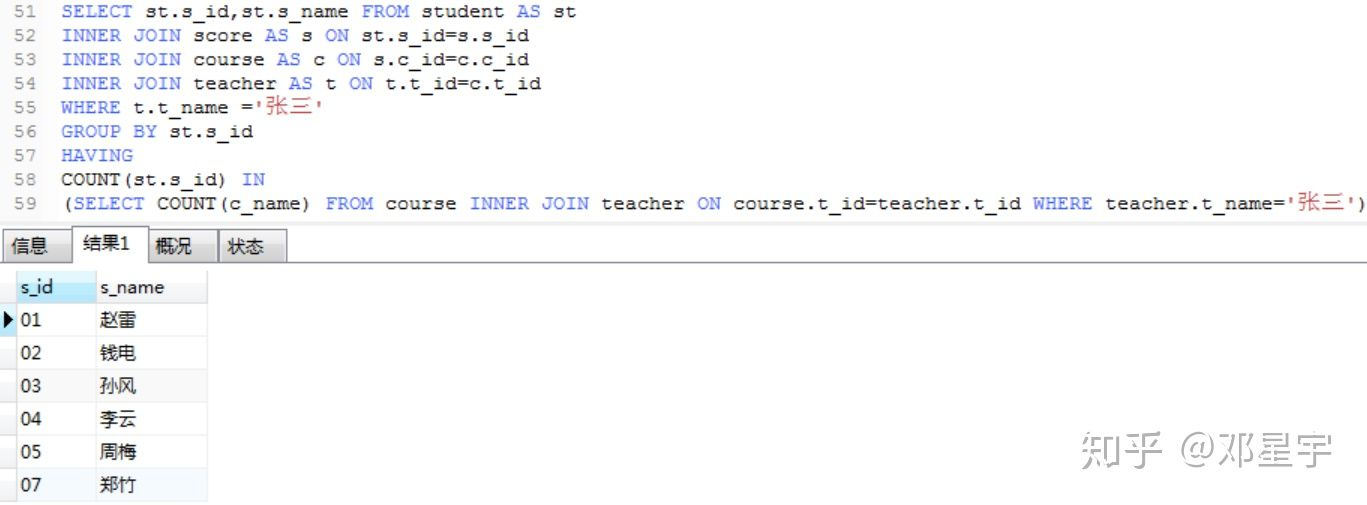-- 7、查询学过编号为“01”的课程并且也学过编号为“02”的课程的学生的学号、姓名

SELECT s_id,s_name FROM student
WHERE s_id IN
(
SELECT a.s_id FROM
(SELECT s_id FROM score WHERE c_id='01')AS a
INNER JOIN
(SELECT s_id FROM score WHERE c_id='02')AS b
ON a.s_id=b.s_id
)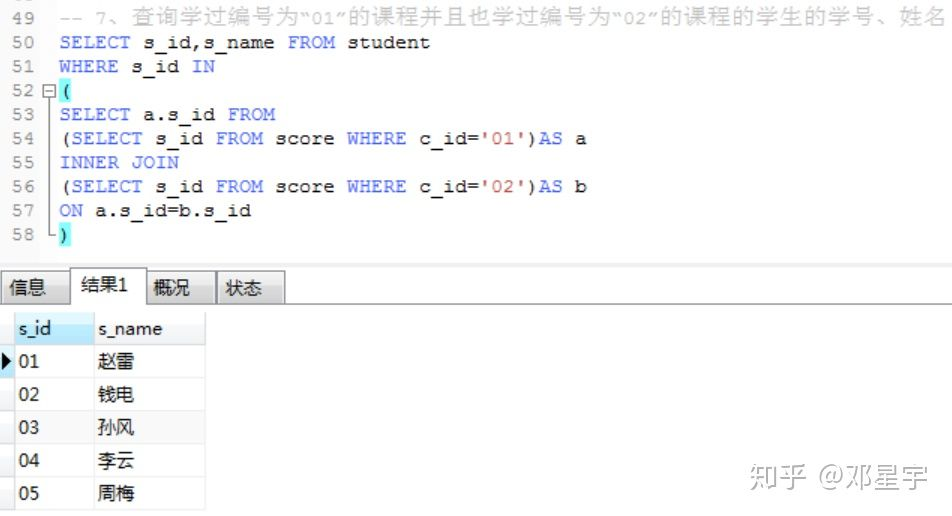SELECT s_id,s_name FROM student
WHERE s_id IN
(SELECT s_id FROM score WHERE c_id='01')
AND s_id IN
(SELECT s_id FROM score WHERE c_id='02')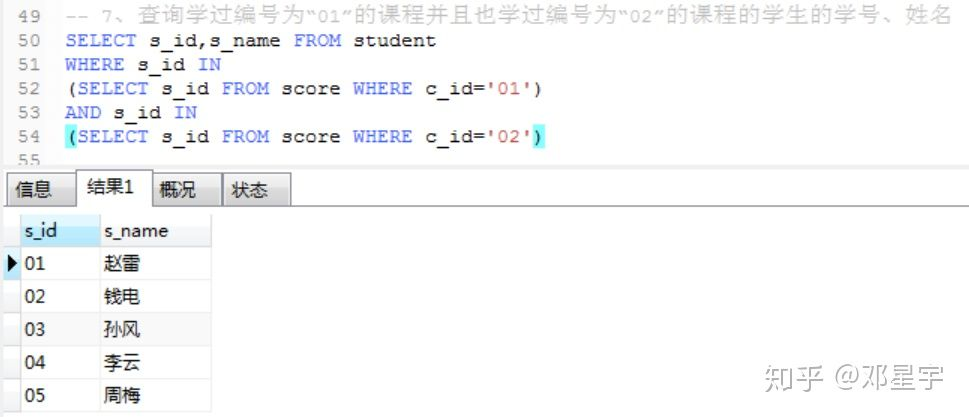-- 8、查询各门的总成绩、平均成绩和人数

SELECT c_id '课程编号',SUM(s_score) '总成绩',
AVG(s_score ) '平均成绩',COUNT(s_score) '人数'
FROM score
GROUP BY c_id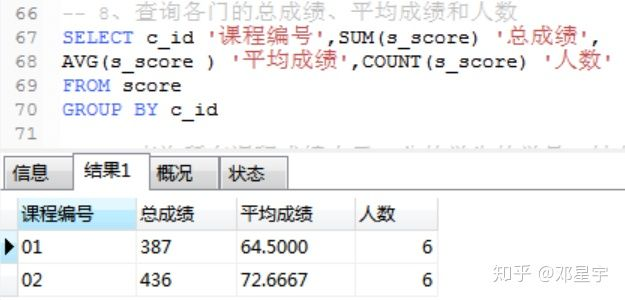-- 9、查询所有课程成绩小于60分的学生的学号、姓名

SELECT a.s_id,t.s_name FROM
(
SELECT s_id,COUNT(c_id) 'count'
FROM score
WHERE s_score<60
GROUP BY s_id
)AS a
INNER JOIN
(
SELECT s_id,COUNT(c_id) 'count'
FROM score
GROUP BY s_id
)AS b ON a.s_id=b.s_id
INNER JOIN student AS t ON a.s_id=t.s_id
WHERE a.count=b.count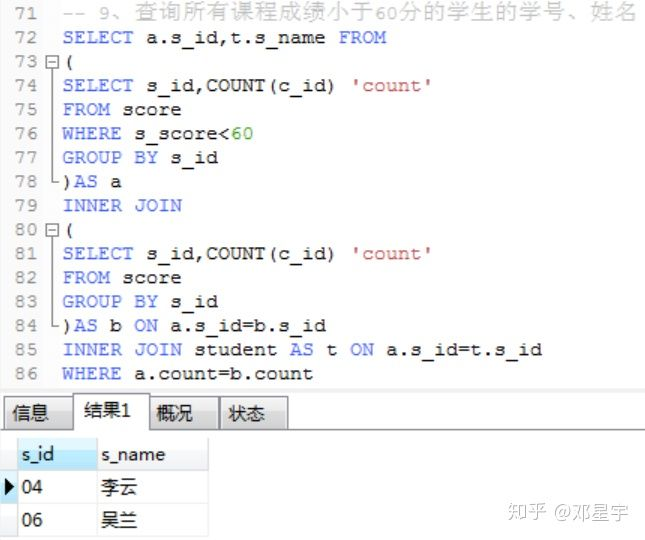-- 10、查询没有学全所有课的学生的学号、姓名

SELECT st.s_id '学生学号',st.s_name '学生姓名'
FROM student AS st
LEFT JOIN score AS sc ON st.s_id=sc.s_id
GROUP BY st.s_id
HAVING COUNT(DISTINCT sc.c_id)<(SELECT count(DISTINCT c_id) FROM course)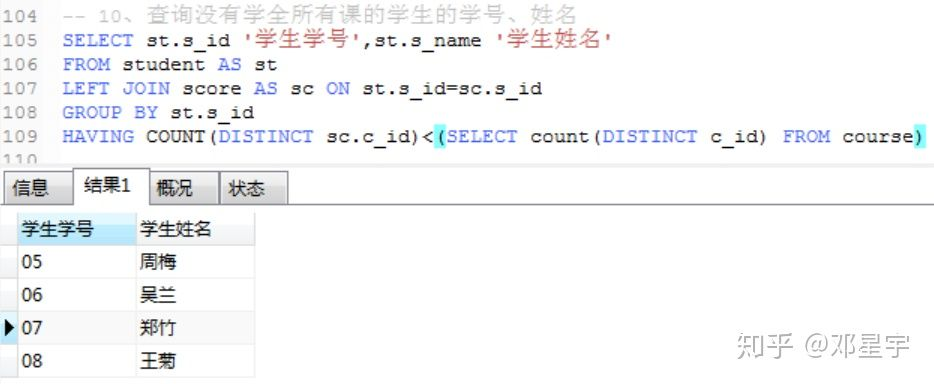-- 11、查询至少有一门课程与学号为“01”的学生所学课程相同的其他同学的学号

SELECT s_id,s_name FROM student
WHERE s_id in
(
SELECT DISTINCT s_id FROM score
WHERE c_id in
(
SELECT c_id FROM score WHERE s_id='01'
)
AND s_id!='01'
)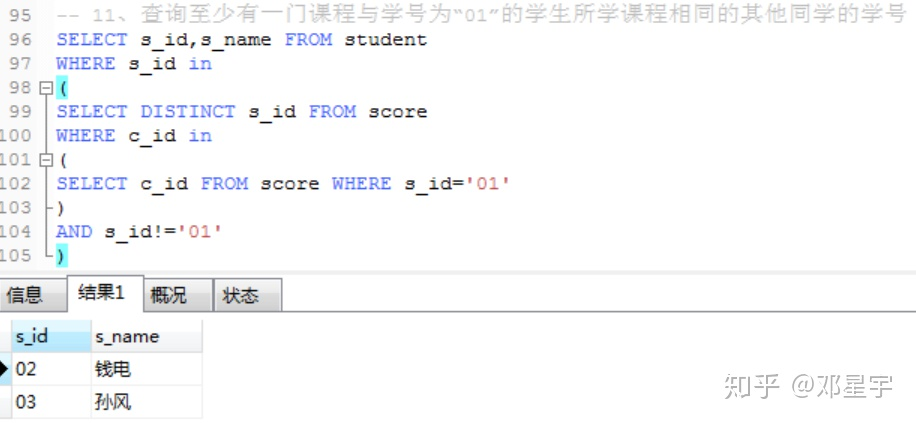-- 12、查询和“01”号同学所学课程完全相同的其他同学的学号

SELECT s_id FROM score
WHERE s_id <>'01'
GROUP BY s_id
HAVING GROUP_CONCAT(c_id)=(
SELECT GROUP_CONCAT(c_id) FROM score WHERE s_id='01')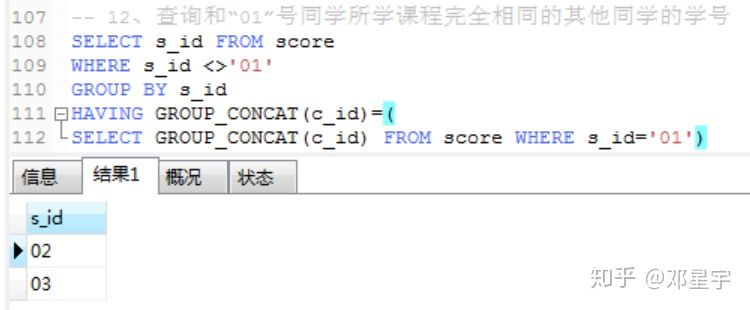SELECT a.s_id FROM
(SELECT * FROM score WHERE s_id!='01') AS a
LEFT JOIN(SELECT * FROM score WHERE s_id='01')AS b
ON a.c_id=b.c_id
GROUP BY a.s_id
HAVING COUNT(b.s_id)=(SELECT COUNT(c_id) FROM score WHERE s_id='01')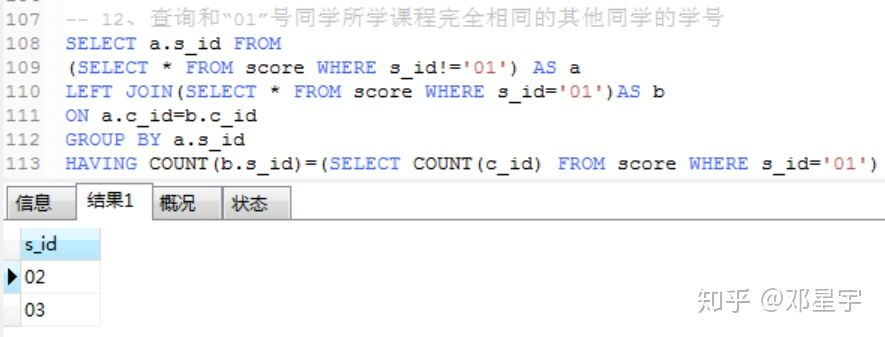-- 15、查询两门及其以上不及格课程的同学的学号，姓名及其平均成绩

SELECT a.s_id,a.s_name,AVG(b.s_score) FROM student AS a
INNER JOIN score AS b
ON a.s_id=b.s_id
WHERE a.s_id IN
(SELECT s_id FROM score AS a
WHERE s_score<60
GROUP BY s_id
HAVING COUNT(s_score)>1)
GROUP BY a.s_id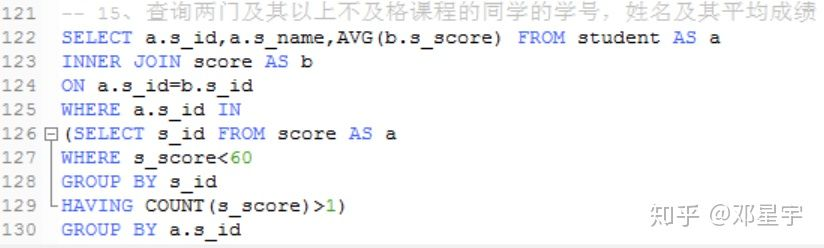-- 16、检索"01"课程分数小于60，按分数降序排列的学生信息

SELECT * FROM student AS a
INNER JOIN score AS b ON a.s_id=b.s_id
WHERE b.c_id='01'
AND b.s_score<60
ORDER BY b.s_score DESC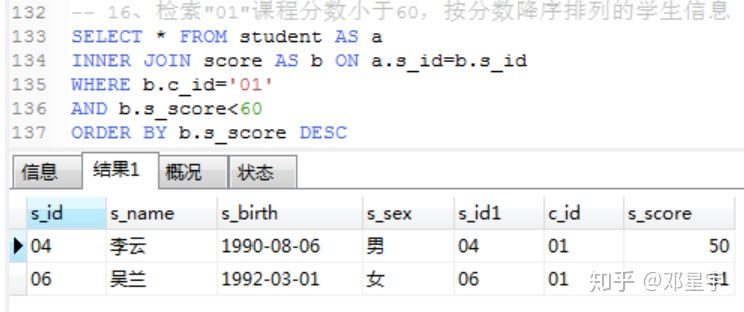-- 17、按平均成绩从高到低显示所有学生的所有课程的成绩以及平均成绩

SELECT
s_id,
MAX(CASE WHEN c_id='01' THEN s_score ELSE NULL END)'01',
MAX(CASE WHEN c_id='03' THEN s_score ELSE NULL END)'02',
MAX(CASE WHEN c_id='02' THEN s_score ELSE NULL END)'03',
AVG(s_score)
FROM score
GROUP BY s_id
ORDER BY AVG(s_score) DESC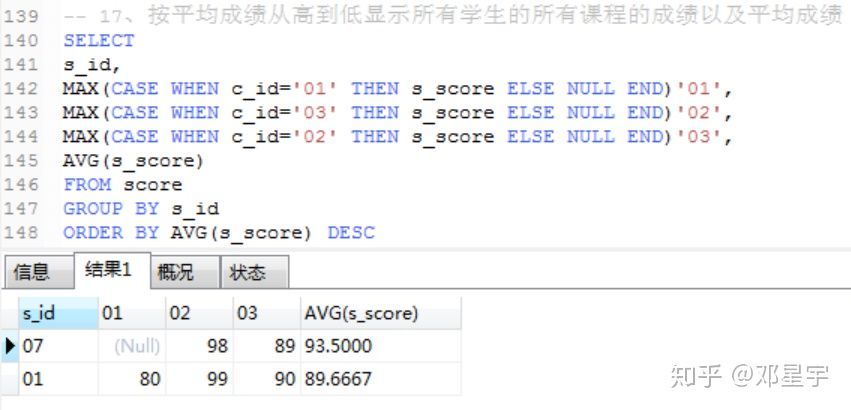-- 18、查询各科成绩最高分、最低分和平均分：以如下形式显示：课程ID，课程name，最高分，最低分，平均分，及格率，中等率，优良率，优秀率

-- 及格为>=60，中等为：70-80，优良为：80-90，优秀为：>=90

SELECT b.c_id,b.c_name,
MAX(a.s_score)'最高分',MIN(a.s_score)'最低分',AVG(a.s_score)'平均分',
SUM(CASE WHEN a.s_score>=60 THEN 1 ELSE 0 END)/COUNT(a.s_id)'及格率',
SUM(CASE WHEN a.s_score>=70 AND a.s_score<80 THEN 1 ELSE 0 END)/COUNT(a.s_id)'中等率',
SUM(CASE WHEN a.s_score>=80 AND a.s_score<90 THEN 1 ELSE 0 END)/COUNT(a.s_id)'优良率',
SUM(CASE WHEN a.s_score>=90 THEN 1 ELSE 0 END)/COUNT(a.s_score)'优秀率'
FROM score AS a
INNER JOIN course AS b ON a.c_id=b.c_id
GROUP BY a.c_id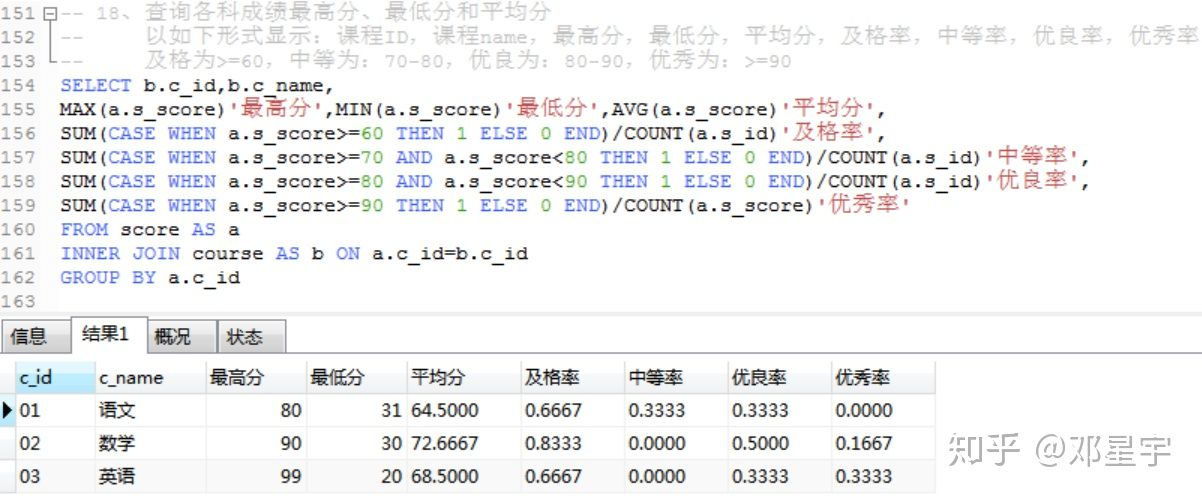-- 19、按各科成绩进行排序，并显示排名

SELECT s_score,rank() over(PARTITION by s_id ORDER BY s_score DESC) FROM score;

-- 20、查询学生的总成绩并进行排名

SELECT *,SUM(s_score) FROM score
GROUP BY s_id
ORDER BY SUM(s_score) DESC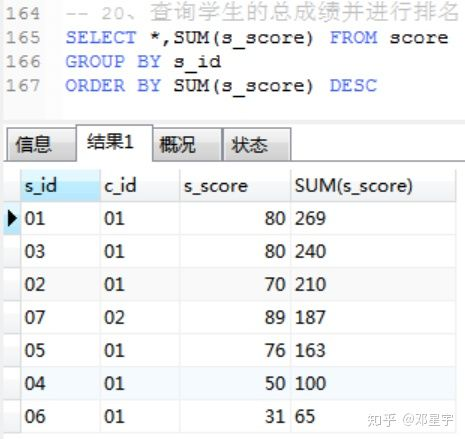-- 21 、查询不同老师所教不同课程平均分从高到低显示

SELECT a.c_id,b.c_name,c.t_name,AVG(a.s_score) FROM score AS a
INNER JOIN course AS b ON a.c_id=b.c_id
INNER JOIN teacher AS c ON b.t_id=c.t_id
GROUP BY a.c_id
ORDER BY AVG(a.s_score) DESC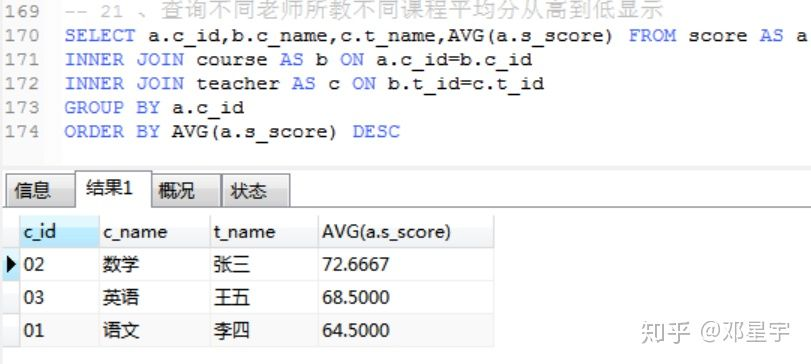-- 22、查询所有课程的成绩第2名到第3名的学生信息及该课程成绩

SELECT * FROM
(
SELECT b.s_id,b.s_name,b.s_birth,,b.s_sex,a.c_id,a.s_score,
row_number()over(PARTITION by a.c_id ORDER BY a.s_score DESC) m
FROM score AS a INNER JOIN student AS b ON a.s_id=b.s_id )
WHERE m IN(2,3)

-- 23、使用分段[100-85],[85-70],[70-60],[<60]来统计各科成绩，分别统计各分数段人数：课程ID和课程名称

SELECT a.c_id,b.c_name,
SUM(CASE WHEN a.s_score<60 THEN 1 ELSE 0 END) '不及格',
SUM(CASE WHEN a.s_score>=60 AND a.s_score<70 THEN 1 ELSE 0 END) '及格',
COUNT(CASE WHEN a.s_score>=70 AND a.s_score<85  THEN 1 ELSE NULL END) '良',
COUNT(CASE WHEN a.s_score>=85 THEN 1 ELSE NULL END) '优'
FROM score AS a
INNER JOIN course AS b ON a.c_id=b.c_id
GROUP BY a.c_id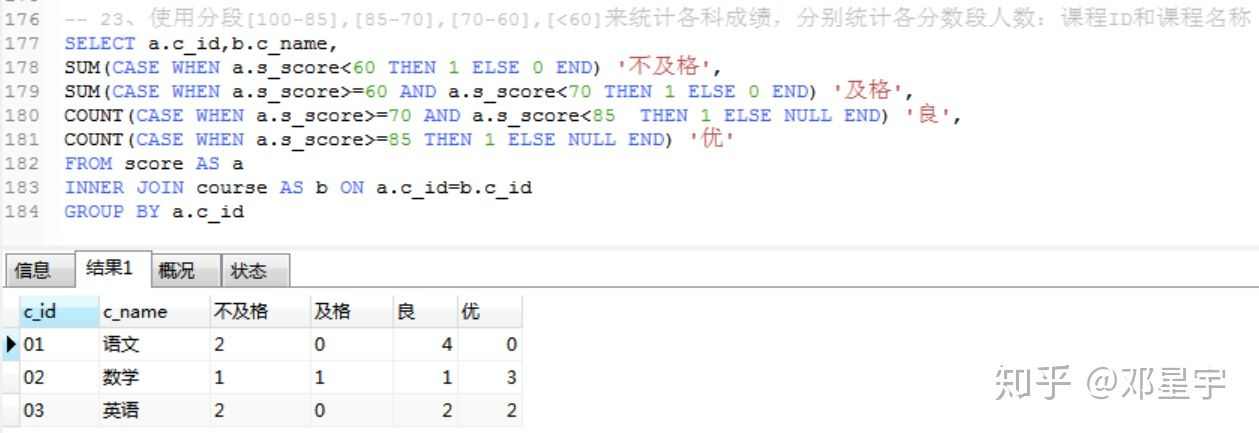-- 24、查询学生平均成绩及其名次

SELECT s_id,AVG(s_score),rank() over(PARTITION by s_id ORDER BY AVG(s_score)DESC) FROM score

-- 25、查询各科成绩前三名的记录（不考虑成绩并列情况）

SELECT *
FROM (SELECT b.s_id,b.s_name,b.s_birth,,b.s_sex,a.c_id,a.s_score,
row_number()over(PARTITION by a.c_id ORDER BY a.s_score DESC) m
FROM score AS a INNER JOIN student AS b ON a.s_id=b.s_id ) a
WHERE m IN(1,2,3)

-- 26、查询每门课程被选修的学生数

SELECT c_id,COUNT(c_id) FROM score
GROUP BY c_id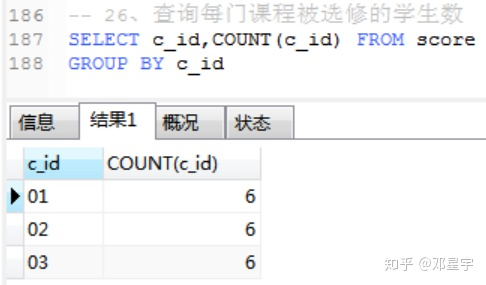-- 27、查询出只有两门课程的全部学生的学号和姓名

SELECT a.s_id,b.s_name,COUNT(a.c_id) FROM score AS a
LEFT JOIN student AS b ON a.s_id=b.s_id
GROUP BY a.s_id
HAVING COUNT(a.c_id)=2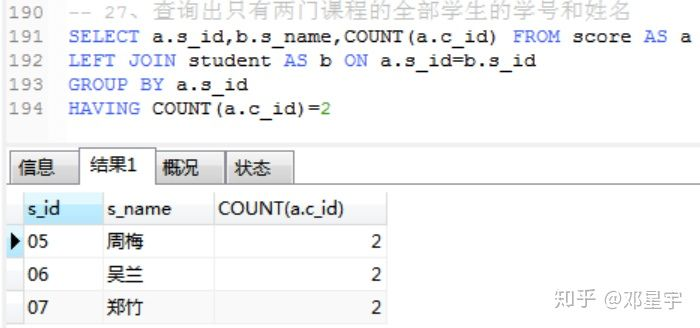SELECT s_id,s_name FROM student
WHERE s_id IN(
(SELECT s_id FROM score
GROUP BY s_id
HAVING COUNT(c_id)=2)
)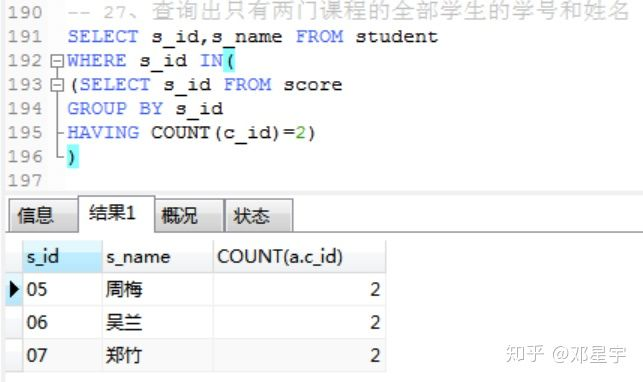-- 28、查询男生、女生人数

SELECT s_sex,COUNT(s_sex)
FROM student
GROUP BY s_sex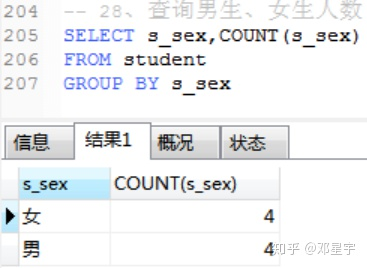SELECT
SUM(CASE WHEN s_sex='男' THEN 1 ELSE 0 END)'男生个数',
count(CASE WHEN s_sex='女' THEN 1 ELSE NULL END) '女生个数'
FROM student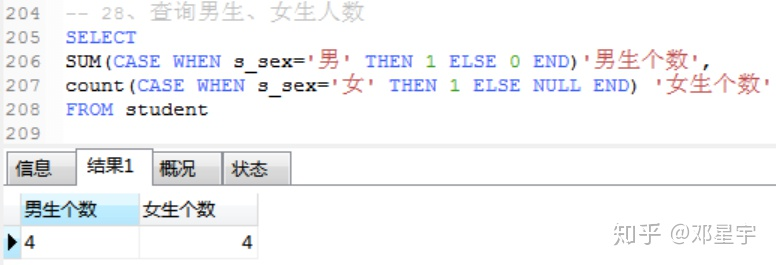-- 29 查询名字中含有"风"字的学生信息

SELECT * FROM student
WHERE s_name LIKE '%风%'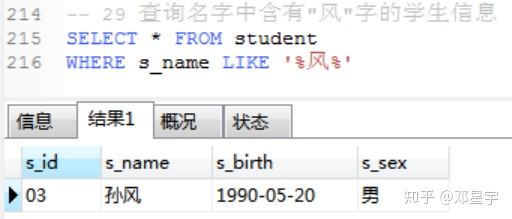-- 31、查询1990年出生的学生名单

SELECT * FROM student
WHERE s_birth LIKE '1990%'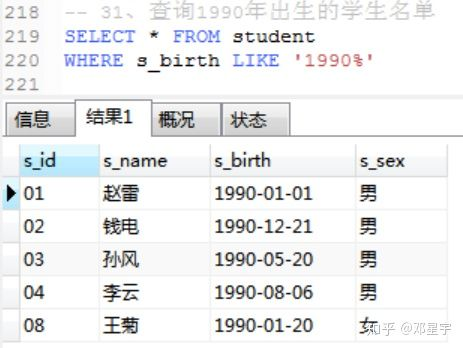SELECT * FROM student
WHERE YEAR(s_birth)=1990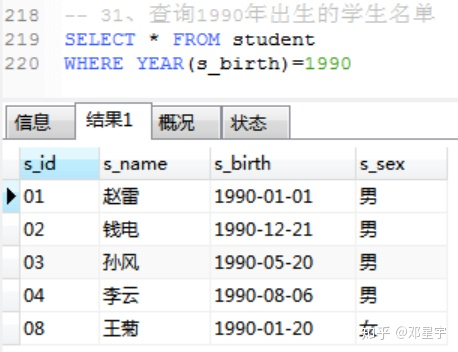-- 32、查询平均成绩大于等于85的所有学生的学号、姓名和平均成绩

SELECT a.s_id,a.s_name,AVG(b.s_score) FROM student AS a
INNER JOIN score AS b ON a.s_id=b.s_id
GROUP BY b.s_id
HAVING AVG(b.s_score)>=85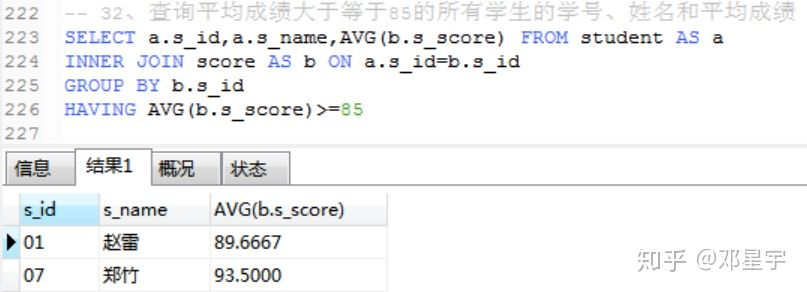-- 33、查询每门课程的平均成绩，结果按平均成绩升序排序，平均成绩相同时，按课程号降序排列

SELECT c_id,AVG(s_score)
FROM score
GROUP BY c_id
ORDER BY AVG(s_score),c_id DESC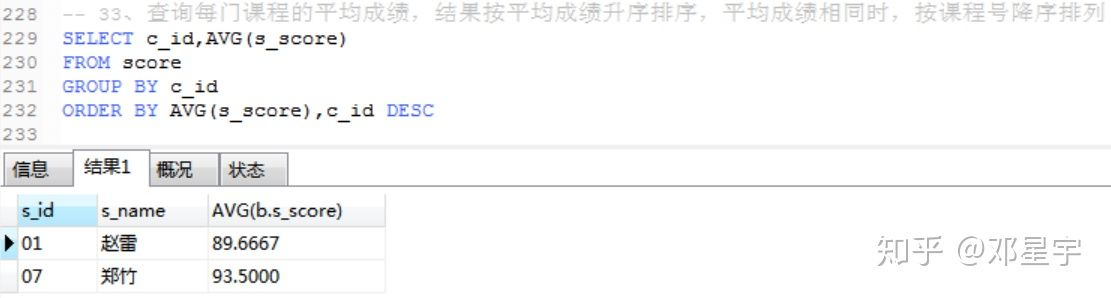-- 34、查询课程名称为"数学"，且分数低于60的学生姓名和分数

SELECT a.s_name,b.s_score FROM student AS a
LEFT JOIN score AS b
ON a.s_id=b.s_id
LEFT JOIN course AS c
ON b.c_id=c.c_id
WHERE c.c_name='数学'
AND b.s_score<60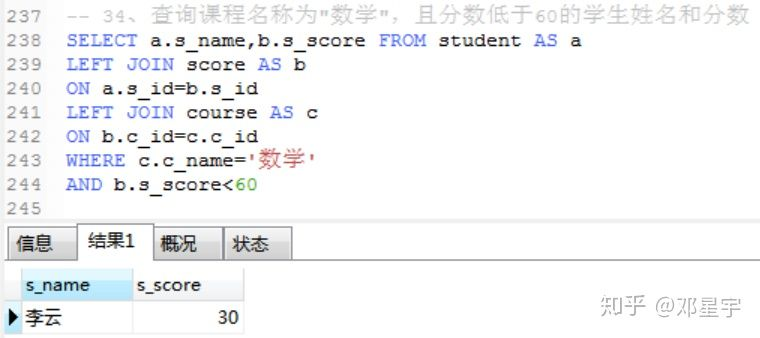-- 35、查询所有学生的课程及分数情况

SELECT a.s_id'学号',a.s_name'姓名',d.s_score'语文',e.s_score'数学',f.s_score'英语'
FROM student AS a
LEFT JOIN
(SELECT b.s_id,c.c_name,b.s_score FROM score AS b
LEFT JOIN course AS c ON b.c_id=c.c_id WHERE c_name='语文')AS d
ON a.s_id=d.s_id
LEFT JOIN
(SELECT b.s_id,c.c_name,b.s_score FROM score AS b
LEFT JOIN course AS c ON b.c_id=c.c_id WHERE c_name='数学')AS e
ON a.s_id=e.s_id
LEFT JOIN
(SELECT b.s_id,c.c_name,b.s_score FROM score AS b
LEFT JOIN course AS c ON b.c_id=c.c_id WHERE c_name='英语')AS f
ON a.s_id=f.s_id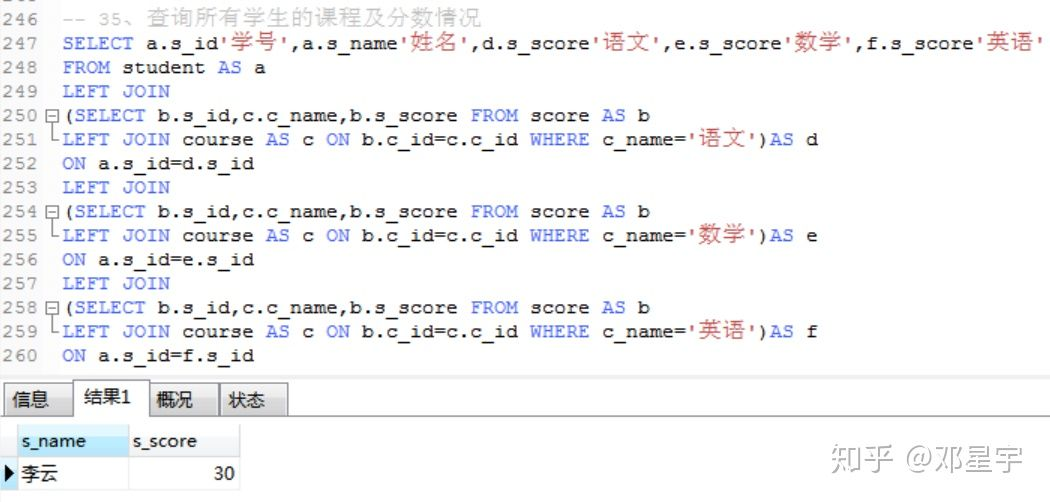SELECT c.s_id,c.s_name,
MAX(CASE WHEN b.c_name='语文' THEN a.s_score ELSE NULL END)'语文',
MAX(CASE WHEN b.c_name='数学' THEN a.s_score ELSE NULL END)'数学',
MAX(CASE WHEN b.c_name='英语' THEN a.s_score ELSE NULL END)'英语'
FROM score AS a
INNER JOIN course AS b ON a.c_id=b.c_id
INNER JOIN student AS c ON a.s_id=c.s_id
GROUP BY c.s_id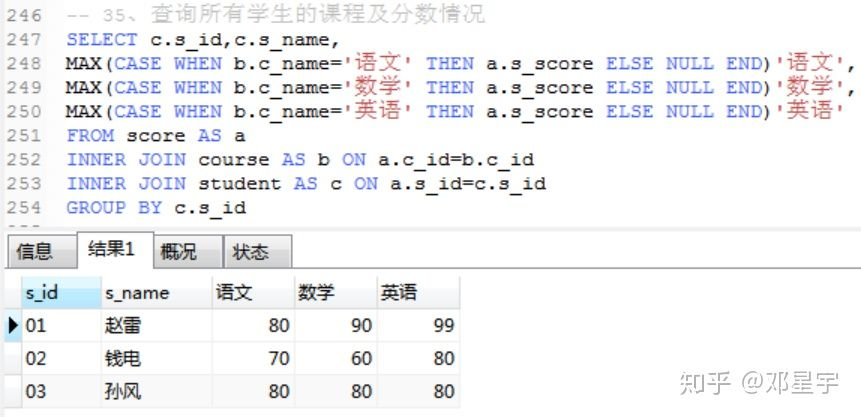-- 36、查询课程成绩在70分以上的学生姓名、课程名称和分数

SELECT a.s_name,c.c_name,b.s_score FROM student AS a
LEFT JOIN score AS b ON a.s_id=b.s_id
LEFT JOIN course AS c ON b.c_id=c.c_id
WHERE b.s_score>70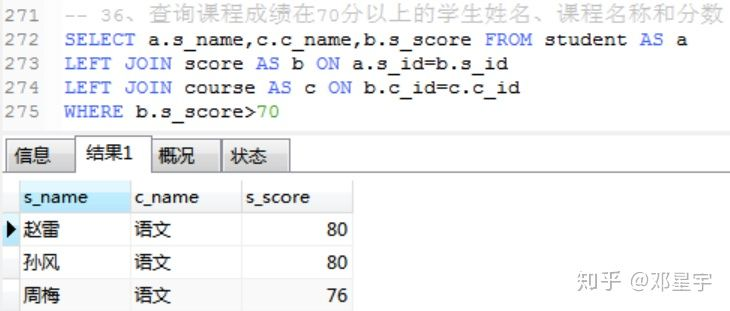-- 37、查询不及格的课程并按课程号从大到小排列

SELECT a.s_id,b.s_name,a.c_id,a.s_score FROM score AS a
INNER JOIN student AS b
ON a.s_id=b.s_id
WHERE a.s_score<60
ORDER BY a.c_id DESC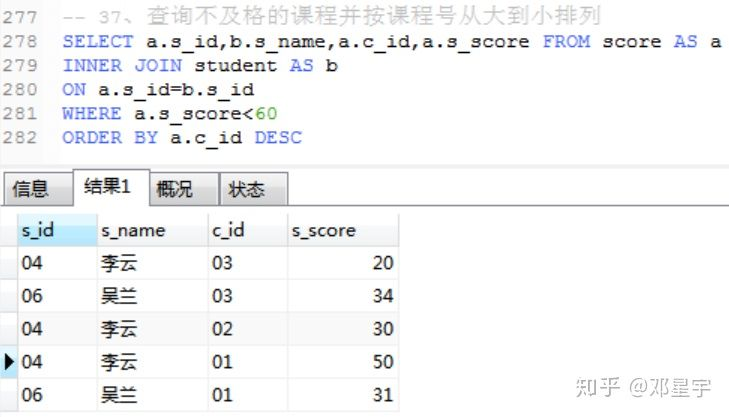-- 38、查询课程编号为03且课程成绩在80分以上的学生的学号和姓名

SELECT a.s_id,b.s_name,a.s_score FROM score AS a
INNER JOIN student AS b
ON a.s_id=b.s_id
WHERE a.s_score>80
AND a.c_id='03'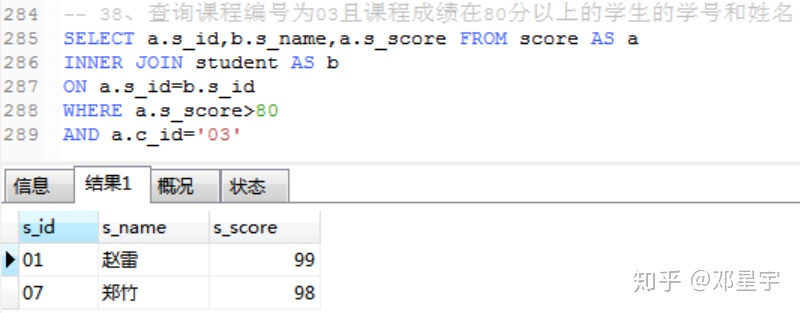-- 39、求每门课程的学生人数

SELECT c_id,COUNT(s_score) FROM score
GROUP BY c_id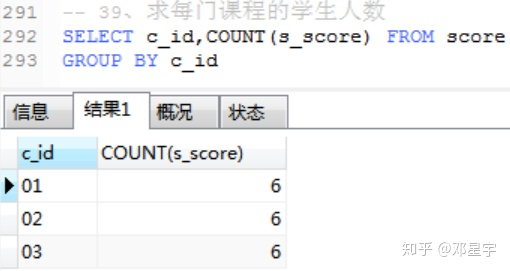-- 40、查询选修“张三”老师所授课程的学生中成绩最高的学生姓名及其成绩

-- 41.查询不同课程成绩相同的学生的学生编号、课程编号、学生成绩

SELECT s_id FROM
(SELECT a.s_id,a.s_score FROM score AS a
INNER JOIN
(SELECT s_id FROM score
GROUP BY s_id HAVING COUNT(DISTINCT c_id)>1)AS b
ON a.s_id=b.s_id
GROUP BY a.s_id,a.s_score
)AS c
GROUP BY s_id HAVING COUNT(s_id)=1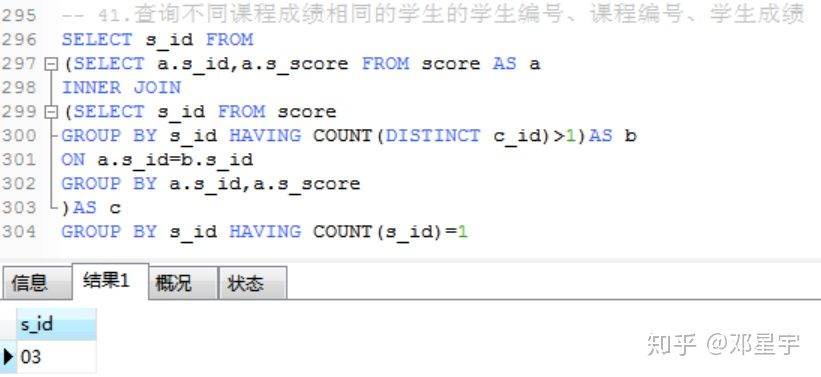-- 43、统计每门课程的学生选修人数（超过5人的课程才统计）。

-- 要求输出课程号和选修人数，查询结果按人数降序排列，若人数相同，按课程号升序排列

SELECT c_id,COUNT(s_score) FROM score
GROUP BY c_id
HAVING COUNT(s_score)>5
ORDER BY COUNT(s_score) DESC,c_id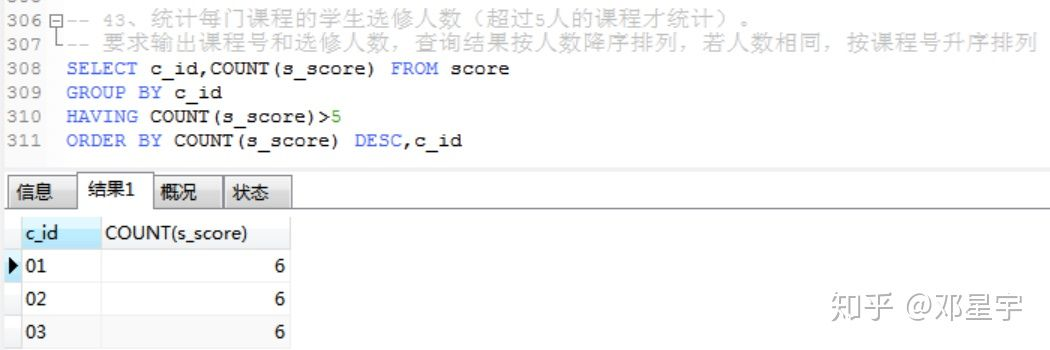-- 44、检索至少选修两门课程的学生学号和选课数

SELECT s_id,COUNT(s_score) FROM score
GROUP BY s_id
HAVING COUNT(s_score)>=2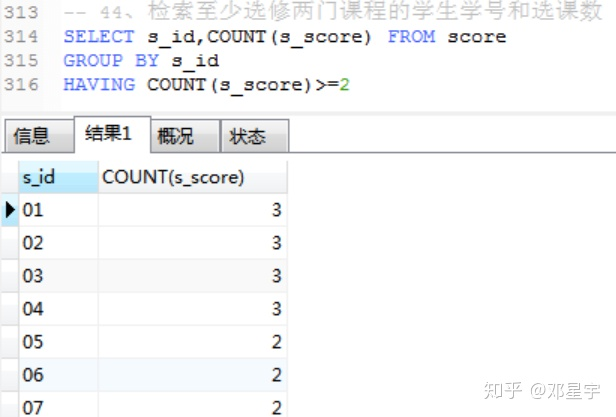-- 45、 查询选修了全部课程的学生信息

SELECT * FROM student AS a
LEFT JOIN score AS b
ON a.s_id=b.s_id
GROUP BY a.s_id
HAVING COUNT(DISTINCT c_id) =( SELECT COUNT(c_id) FROM course)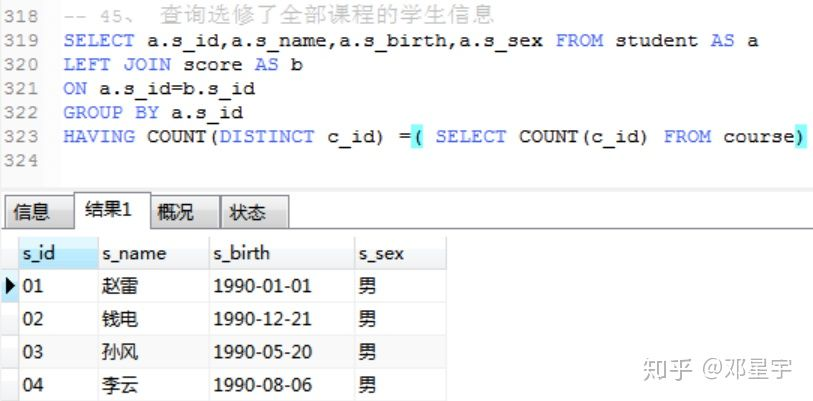-- 46、查询各学生的年龄（精确到月份）

SELECT s_id,s_birth,DATEDIFF('2020-5-20',s_birth)/365 FROM student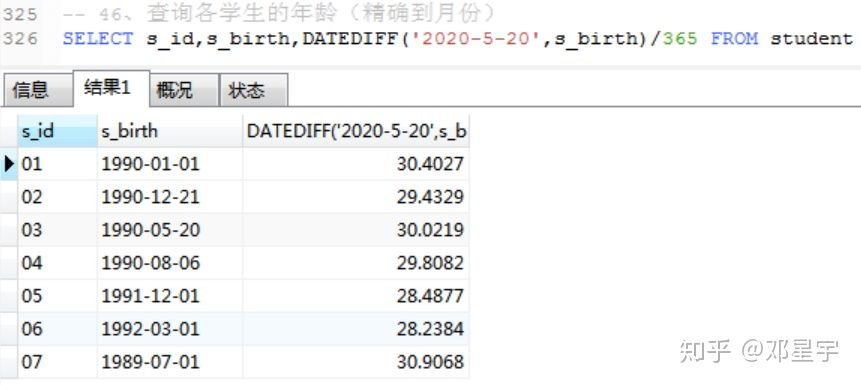-- 50、查询下个月过生日的学生

SELECT * FROM student WHERE
MONTH(s_birth)=MONTH(date(NOW()))%12+1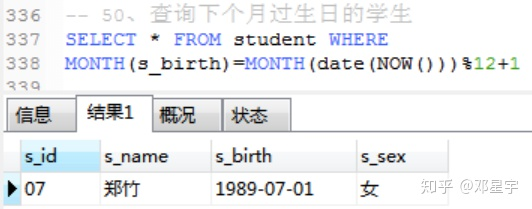## 知识点：

1.inner jion、right jion、left jion的区别

inner join为“有效的连接”，就是根据on后面的关联条件，两张表中都有的数据才会显示

left join为主表全显示，连接后的表看on后面的选择条件，left join后面的条件，并不会影响左表的数据显示，左表数据会全部显示出来，连接的表如果没有数据，则全部显示为null

right join为“主表看on,后表全显”(右表数据不受影响)，即右表数据全部显示，主表数据看on后面的选择条件

2.字符匹配

_代表一个字符， %代表0个及以上字符

3.rank，dense_rankrow_number的区别

rank(跳跃排序)

92 1

82 2

82 2

67 4

dense_rank（连续排序）

92 1

82 2

82 2

67 3

row_number（无重复值排序）

92 1

82 2

82 3

67 4

1、插入数据：

insert into 表名 values( ) #输入的值要跟原表对应

insert into Teacher values('02' , '李四')

2、查询表中的数据：

select * from 表名 # *号代表取全部列的数据

select * from student

select 指定列 from 表名

SELECT t_id,s_score FROM score

select avg(列名) from 表名

SELECT c_id '课程编号',SUM(s_score) '总成绩',AVG(s_score ) '平均成绩',COUNT(s_score) '人数' FROM score

GROUP BY c_id

select 指定列 from 表名 where 条件

SELECT s_id FROM score WHERE s_id <>'01'

（1）>大于 ；<小于； =等于； <>和!=不等于

（2）like 字符匹配 select * from teacher where t_name like '张%'

（3）between...and 区间 SELECT * FROM score WHERE s_score BETWEEN 70 AND 90

（4）in 指定数据集作为条件，也常用于子查询

3、GROUP BY 语句

SELECT 列名或统计量 FROM 表名 GROUP BY 列名

SELECT c_id,SUM(s_score),AVG(s_score ),COUNT(s_score)

FROM score

GROUP BY c_id

GROUP BY后的条件查询用having

4、ORDER BY 语句

SELECT *,SUM(s_score) FROM score GROUP BY s_id ORDER BY SUM(s_score) DESC

5、year、month、day、now语句

SELECT * FROM student WHERE YEAR(s_birth)=1990；

SELECT * FROM student WHERE MONTH(s_birth)=01；

SELECT * FROM student WHERE DAY(s_birth)='01'；

2020-05-20、20200520、2020/05/20

now()

6、LIMIT语句

SELECT c_id FROM score

LIMIT 0，1 #表示从0开始（第一行开始），选取第一行数据

7、DATEDIFF语句

SELECT s_id,s_birth,DATEDIFF('2020-5-20',s_birth)/365 FROM student

11-2701-104358
06-174358
04-023289
03-164005
05-233106
06-262146
09-194万+
04-13560
05-169万+
07-283285
04-254万+
10-102万+
05-101085
01-201732
11-27
10-183169点击重新获取扫码支付余额充值